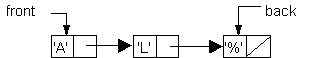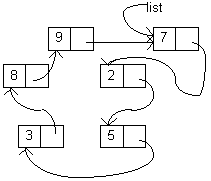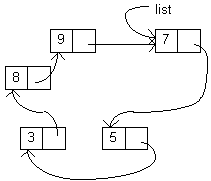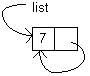Making a Circular Queue

One of the nice applications of pointers is in implementing a circular queue. First let us consider the fact, that we only need one pointer and that is to the last element in the queue.

In the example below, the node containing 2 is the head of the list and the node containing 9 is the rear of the list.To enQueue a node containing 7 the queue would look like this:And then if we were to `deQueue` a node, we would dequeue the node containing the 2 and the result would look like this.In this implementation, an empty list would be `list = NULL`.

A list containing one element would look like this. In coding enQueue, care must be taken with the first element.To get rid of a queue, or `makeEmpty`, the nodes must be disposed so that the memory is free again.

Use the `myqueue` Class, a pointer implementation of a queue, to modify the code to be a circular queue.

In your client program, to show that each function works, write a non-destructive function show that will display the contents of the `queue`.

Continue to:  Unit 7 / Prev / Next